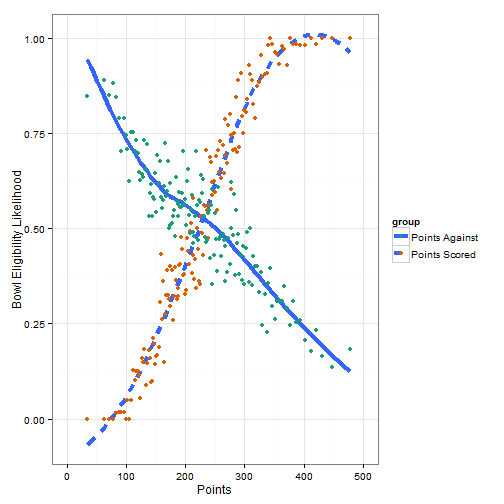## Offense or defense improve likelihood of becoming bowl eligible?

I saw a post recently about the likelihood of a baseball team winning based on how many runs, hits, and other baseball statistics. I liked the idea and thought of applying that to college football. Particularly, I’m interested in knowing whether scoring more points or having a stout defense improves the likelihood of becoming bowl eligible.

Using some data scraped from the cfbDatawarehouse to figure out how likely a team would be bowl eligible based on the number of points they score. Below are the results of my exploration looking at both the points scores metric and the points against metric (also there is a quick interactive rCharts version of the plot down further). Seems to me that scoring more points leads to more assured success in the college game, perhaps that is why offensive recruits seem to be more sought after. This would also be interesting to create by decade to see if trends have shifted over the years.

Enjoy, plots (and code for plots) shown below.

``````p <- ggplot(coaches, aes(x = PF)) + theme_bw()
p + stat_smooth(data = ovrBE, aes(x = avg, y = bpct, linetype = group),
se = FALSE, size = 1.5, method = "loess") +
geom_point(data = ovrBE, aes(x=avg, y = bpct, color = group), size = 2) +
scale_x_continuous("Points", limits = c(0, 500), breaks = c(0, 100, 200, 300, 400, 500)) +
scale_color_brewer(palette = "Dark2") +
ylab("Bowl Eligibility Likelihood")
````````````library(rCharts)

h1 <- hPlot(x = "avg", y = "bpct", group = "group", data = ovrBE)
h1\$yAxis(title = list(text = "Bowl Eligibility Likelihood"), min = 0, max = 1, tickInterval = .1)
h1\$xAxis(title = list(text = "Points"),
min = 0, max = 500, tickInterval = 100)
h1\$legend(verticalAlign = "top", align = "right", layout = "vertical", title = list(text = "Group"))
h1\$plotOptions(series = list(lineWidth = 2))
h1\$print('chart1', include_assets = TRUE, cdn = TRUE)
``````